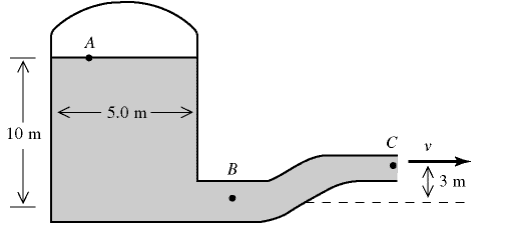# Pressure in tank pipe -- Bernoulli Equations

## Homework Statement[/B]
A pressurized cylindrical tank, 5m in diameter, contains water which emerges from the pipe at point C with a velocity of 25 m/s. Point A is 10m above point B and point C is 3m above point B. The area of the pipe at point B is 0.07 m2 and the pipe narrows to an area of 0.02m2 at Point C. Assume the water is an ideal fluid in laminar flow. The density of water 1000kg/m3

## Homework Equations

Continuity Equation:
VBAB=VCAC

Bernouli's equation:
Let D = density of water
PB + (1/2)DVB2+DghB = PC + (1/2)DVC2+DghC

## The Attempt at a Solution

Let D = density of water
VBAB=VCAC
Therefore
VB=[VCAC]/AB=[25*0.02]/0.07=7.14m/s

PB + (1/2)DVB2+DghB = PC + (1/2)DVC2+DghC
Therefore
PB = PC + (1/2)DVC2+DghC -(1/2)DVB2-DghB

Except PC and PBis unknown so this approach shouldn't work. One of my peers claims it is the same as 1 ATM but I'm doubtful since this does not result in answer which is accurate. I don't see how The pressure at Point C could possibly be 1atm... 2 unknowns .. 1 equation.. not going to work :( correct me if wrong.

Upon searching the internet I came across the solution.. which does yield what I firmly believe is the correct answer .. however I don't not understand how this formula came about..

Let D = density of water

PB = [VC2/(2G) - VB2/(2G) + y)Dg
=(252/(2*9.8) - (7.142/(2*9.8) + 3)*1000*9.8
~=~ 316410 Pa ~=~ 320 kPa

It appears to be some variation of the Bernoulli formula but I need to demonstrate how to take those formulas and make them into this format... which I don't even know where to begin. I don't need to know how to derive the formulas that this one came from .. in other words.. I can just say here's the Bernoulli equation .. rearrange and combine like so and obtain this: (without having to derive the Bernoulli equation itself).

What are you trying to find? Pressure at B? Or Pressure at A? You don't need to consider what's going on at point B to do that.

Except PC and PBis unknown so this approach shouldn't work. One of my peers claims it is the same as 1 ATM but I'm doubtful since this does not result in answer which is accurate. I don't see how The pressure at Point C could possibly be 1atm...
.
Presumably the pipe vents to atmosphere, so point C must be at atmospheric pressure.

Let D = density of water

PB = [VC2/(2G) - VB2/(2G) + y)Dg
=(252/(2*9.8) - (7.142/(2*9.8) + 3)*1000*9.8
~=~ 316410 Pa ~=~ 320 kPa

It appears to be some variation of the Bernoulli formula but I need to demonstrate how to take those formulas and make them into this format... which I don't even know where to begin. I don't need to know how to derive the formulas that this one came from .. in other words.. I can just say here's the Bernoulli equation .. rearrange and combine like so and obtain this

That is just bernoulli's equation, you were actually very close to reaching that arrangement :
.
PB = PC + (1/2)DVC2+DghC -(1/2)DVB2-DghB

Use gauge pressure as your datum so PC = 0, and let your height datum be at point B so hB = 0. Plug all those in and collect like terms and you'll reach the solution you quoted, note that the answer is still guage, not absolute pressure.

•What are you trying to find? Pressure at B? Or Pressure at A? You don't need to consider what's going on at point B to do that.

Presumably the pipe vents to atmosphere, so point C must be at atmospheric pressure.

That is just bernoulli's equation, you were actually very close to reaching that arrangement :
.

Use gauge pressure as your datum so PC = 0, and let your height datum be at point B so hB = 0. Plug all those in and collect like terms and you'll reach the solution you quoted, note that the answer is still guage, not absolute pressure.
Changed it to 0 and bam same kPa in for gauge pressure. Thanks a ton you have no idea how many countless hours I spent on this damn problem.

Changed it to 0 and bam same kPa in for gauge pressure. Thanks a ton you have no idea how many countless hours I spent on this damn problem.
Great, you're welcome.